# JEE Main Applications of Integration Previous Year Questions With Solutions

JEE Previous year questions and solutions on the Chapter application of integrations are available here. Application of Integrals is applied in various fields like Engineering, Mathematics, Science, etc. In the field of Mathematics, integrals are used to find the areas under curves, arc length of a curve and surface area, areas bounded by a curve, average value of a curve, area between curves, surface area and the volume of geometric solids and many more. Students get to learn the problem-solving method of the questions present in this concept, revolving around the concepts mentioned above, by practicing the application of integral questions provided underneath. We have prepared Chapter wise solutions for the questions asked in past year JEE exams to help students to check their preparation level.

## JEE Main Past Year Questions With Solutions on Application of Integrations

Question 1: Find the area of the region A = {(x,y) Є R x R| 0 ≤ x ≤ 3 and 0 ≤ y ≤ 4 and y ≤ x2 + 3}. [area in sq. units]

Solution:

Given: A = {(x,y) Є R x R| 0 ≤ x ≤ 3 and 0 ≤ y ≤ 4 and y ≤ x2 + 3}.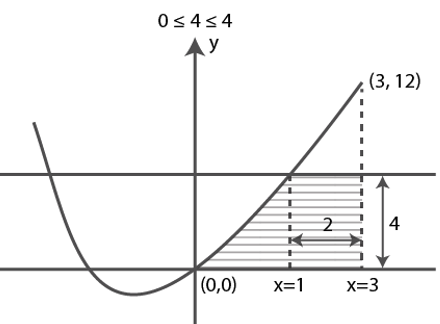Shaded region be required region. So, from above diagram

Required Region = $\int_0^1 (x^2 + 3x) \; dx + (2 \times 4)$

= $(\frac{x^3}{3} + \frac{3x^2}{2})_0^1$ + 8

= 59/6

Question 2: Let S(α) = {(x, y); y2 ≤ x, y2 ≤ α } and, A(α) is area of the region S(α). If for λ, 0 < λ < 4 and A(λ) : A(4) = 2 : 5. Then what will be the value of λ.

Solution:

Let S(α) = {(x, y); y2 ≤ x, y2 ≤ α }

$A(α) = 2 \int_0^{\alpha} \sqrt{x} \; dx = 2 \alpha^{3/2}$

A(4) = 2 x 43/2 = 16 and A(λ) = 2 x λ3/2

As we know,

A(λ)/A(4) = 2/5

On putting values, we have

=> λ = 4 (4/25)1/3

Question 3: Find the area of the region A = {(x, y) ; y2/2 ≤ x ≤ y + 4}.

Solution: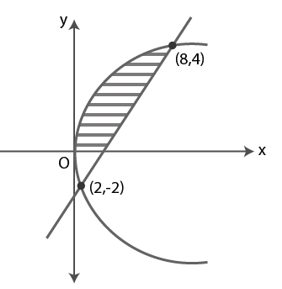Consider equations from the given inequalities,

y2 = 2x and x – y = 4

Here, y2 = 2x is equation of parabola open towards the +ve x-axis and having focus (1/2, 0)

and x – y = 4, is a straight line.

Solving above equations, we get

y2 = 2(y + 4)

or y2 – 2y – 8 = 0

or y = 4 or y = -2

Therefore, point of intersection of line and curve are (2, -2) and (8, 4).

Required Area = $\int_{-2}^4 [(y + 4) – \frac{y^2}{2}]dy$

= $[\frac{(y+4)^2}{2}]_{-2}^4 – \frac{1}{2} [\frac{y^3}{3}]_{-2}^4$

= 1/2[64 – 4] – 1/6(64 + 8)

= 18 sq. units.

Question 4: Find the area of the region bounded by the curves y = |x + 1| and y = 2x, in the 1st quadrant.

Solution:

Given curves are y = |x + 1| and y = 2x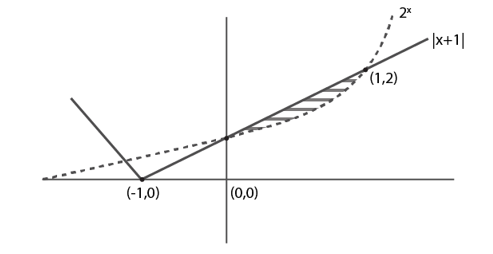From diagram,

Required area = $\int_0^1[(x + 1) – 2^x] \; dx$

= $[\frac{x^2}{2} + x – \frac{2^x}{log_e 2}]_0^1$

= 3/2 – 1/loge2

Question 5: Find the value of λ if area bounded by the parabola y2 = 4λ x and line y = λx is 1/9. Here λ > 0.

Solution:

Parabola y2 = 4λ x and line y = λx

=> (λx)2 = 4λx

=> λ2 x2 = 4λx

Solving above equation ,we get

x = 0 and x = 4/λ

Now, required area is

= $\int_0^{4/\lambda} (\sqrt{4 \lambda x} – \lambda x) \; dx = 1/9$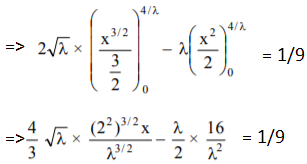=> 32/3λ – 8/λ = 1/9

=> λ = 24

Therefore, the value of λ is 24. Answer!

Question 6: Find the area bounded by the parabola y = x2 – 1, the tangent at the point (2, 3) to it and the y-axis.

Solution: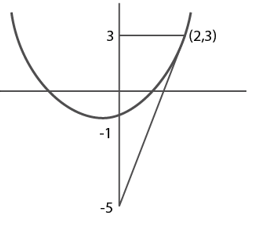Equation of parabola y = x2 – 1

Tangent at (2, 3) is (y + 3)/2 = 2x – 1

or y = 4x – 5

From diagram, required area is

= $\int_{-5}^3(\frac{y+5}{4}) \; dy – \int_{-1}^3 \sqrt{y+1} \; dy$

= $[\frac{y^2/2 + 5y}{4}]_{-5}^3 – \frac{2}{3}[(y+1)^{3/2}]_{-1}^3$

on solving above expression, we have

Require area = 8/3 sq. units.

Question 7: Find the area (in sq. units) of the region A = {x, y) ; 0 ≤ y ≤ x|x| + 1 and -1 ≤ x ≤ 1}.

Solution:

The required area, A is

A = $\int_{-1}^0(-x^2 + 1) dx + \int_{-1}^1(x^2 +1) dx$

= $[\frac{-x^3}{3} + x]_{-1}^0 + [\frac{x^3}{3} + x]_{0}^1$

= 0 – (1/3 – 1) + 1/3 + 1

= 2

Required area is 2 sq. units.

Question 8: Find the value of k, if the area enclosed between the curves x = ky2 and y = kx2, k > 0, is one square unit.

Solution:

Given curves are x = ky2 and y = kx2

form given curves, we have

x = k(kx2) 2

=> x = k (k2x4)

=> x = 0 or x3 = (1/k) 2

=> x = 0, 1/k

So, (0, 0) and (1/k, 1/k) are the points of intersection.

Now,

The required area = $\int_0^{1/k} (\sqrt{\frac{x}{k}} – kx^2) \; dx = 1$

=> $(\frac{1}{\sqrt k} \times \frac{x^{3/2}}{3/2} – \frac{kx^3}{3})^{1/k}= 1$

=> 2/3k2 – 1/3k2 = 1

=> 1/k2 = 3

=> k2 = 1/3

or k = 1/√3

Question 9: Find the area (in sq. units) bounded by the curve x2 = 4y and the straight-line x = 4y – 2.

Solution: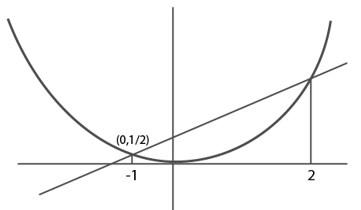First find point of intersection between curve and straight line.

x2 = 4y and x = 4y – 2.

x = x2 – 2

=> x2 – x – 2 = 0

=> (x + 1)(x – 2) = 0

Now, required area = $\int_{-1}^2(\frac{x+2}{4} – \frac{x^2}{4}) \; dx$

= $\frac{1}{4} \int_{-1}^2 (x + 2 – x^2) \; dx$

= $\frac{1}{4}[(x^2/2 + 2x – x^3/3)]_{-1}^2$

= 1/4[(6 – 8/3) – (5/6 – 2)]

= 1/4[10/3 + 7/6]

= 9/8

Question 10: What will be the area in the first quadrant bounded by the parabola, y = x2 + 1, the tangent to it at the point (2, 5) and the coordinate axes.

Solution: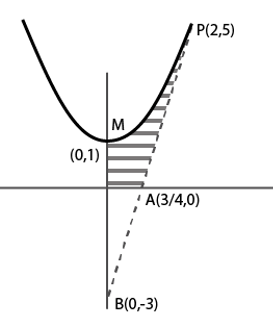The equation of tangent is:

(y + 5)/2 = 2x + 1

=> y + 5 = 4x + 2

or y = 4x – 3

Required area = area of region MBP – Area of triangle AOB

= $\int_0^2$[(x^2 + 1) – (4x – 3)] dx – 1/2 x 3/4 x 3

= $\int_0^2 x^2 – 4x + 4 dx – 9/8$

= $[x^3/3 – 4x^2/2 + 4x ]_0^2 – 9/8$

= 8/3 – 8 – 9/8

= 37/24

Question 11: Find the area of the region bounded by the parabola, y = x2 + 2 and the lines, y = x + 1, x = 0 and x = 3.

Solution:

From the given equations, let us draw the point of intersection and region to find the area.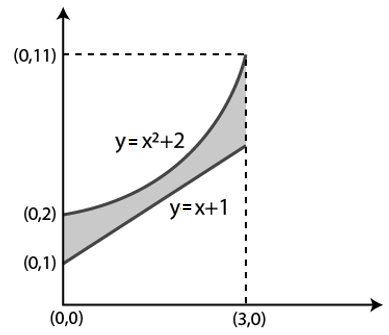Required area = $\int_0^3(x^2 + 2) – (x + 1) \; dx$

= $\int_0^3(x^2 – x + 1) \; dx$

= 15/2

Question 12: The area of the region, enclosed by the circle x2 + y2 = 2 which is not common to the region bounded by the parabola y2 = x and the straight line y = x, is

(a) 1/3(12π – 1)

(b) 1/6(12π – 1)

(c) 1/3(6π – 1)

(d) 1/6(24π – 1)

Solution:

Explanation: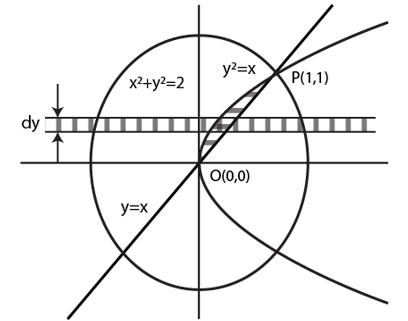Required Area = Area of the circle – Area bounded by given line and parabola

= πr2$\int_0^1$ (y – y^2) dy

= 2π – $(y^2/2 – y^3/3)_0^1$

= 2π – 1/6

= 1/6(12π – 1) sq. units.

Question 13: Find the area of the region {(x, y) Є R | 4x2 ≤ y ≤ 8x + 12} is

(a) 125/3

(b) 128/3

(c) 124/3

(d) 127/3

Solution:

Explanation: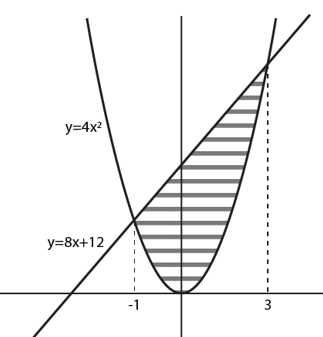For point of intersection,

4x2 = 8x + 12

or x = 3, -1

Area bounded is given as:

A = $\int_{-1}^3(8x + 12 – 4x^2) \; dx$

= $[8x^2 + 12x – 4x^3/3]_{-1}^3$

= 128/3

Question 14: The area bounded by the curve $y\le {{x}^{2}}+3x,0\le y\le 4,0\le x\le 3$ is

(a) $\frac{59}{6}$(b) $\frac{57}{4}$(c) $\frac{59}{3}$(d) $\frac{57}{6}$

$y={{x}^{2}}+3x$ $y=4$ $\Rightarrow {{x}^{2}}+3x=4$ $\Rightarrow {{x}^{2}}+3x-4=0\Rightarrow x=1\,\,or\,\,x=-4$
Area $=\int\limits_{0}^{1}{\left( {{x}^{2}}+3x \right).\,dx+}$ Area + Rectangle
$=\left[ \frac{{{x}^{3}}}{3}+\frac{3{{x}^{2}}}{2} \right]_{0}^{1}+2\left( 4 \right)$ $=\frac{1}{3}+\frac{3}{2}+8=\frac{59}{6}$